# CATV Minimum Loss Pad for 75Ω Measurements

### Abstract

CATV systems are based on 75Ω characteristic impedance, but typical laboratory equipment has 50Ω characteristic impedance. A 75Ω to 50Ω minimum loss pad (MLP) is a broadband method to measure voltage and power accurately. This article describes the resistor network required for such a MLP. Equations are also derived for a MLP with general source and load impedance.

Also see:

### Introduction

Minimum loss pads enable measurement of broadband 75Ω circuits, such as CATV tuner ICs, cable upstream amplifiers, and satellite DBS tuner ICs, using 50Ω test equipment. When working with a MLP, it is imperative that you know whether to use the MLP's voltage loss or power loss. The amount of power loss with a MLP differs from voltage loss due to the impedance transformation. Specifically, power loss, which is used for power measurements, is the same when transforming in either direction. Voltage loss, which is used for voltage measurements, differs depending on the direction of impedance transformation.

### Summary of 75Ω to 50Ω MLP CharacteristicsFigure 1. 75Ω to 50Ω minimum loss pad.

Figure 1 shows a MLP for conversion between 75Ω and 50Ω. The 75Ω termination sees a 75Ω equivalent resistance network. Similarly, the 50Ω termination sees a 50Ω equivalent resistance network.

Table 1 shows that the power loss remains the same when transforming from 75Ω to 50Ω or from 50Ω to 75Ω. The voltage loss, however, is greater when transforming from 75Ω to 50Ω than from 50Ω to 75Ω.

Table 1. 75Ω to 50Ω MLP Power and Voltage Loss

 MLP impedance transformation(Ω to Ω) Power loss (dB) Voltage loss (dB) 75 to 50 -5.72 -7.48 50 to 75 -5.72 -3.96 (50 to 75) + (75 to 50) -11.44 -11.44

### General MLP Resistor Value Equations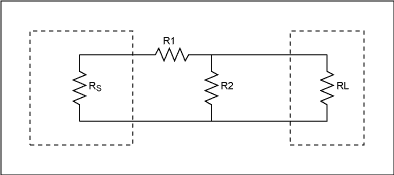Figure 2 shows a MLP with source and load impedances of RS and RL. An L-section pad is used to match the high impedance, RS, to the low impedance, RL. (Figure 2 assumes that RS > RL.) Resistor values for this MLP are determined by Equation 1 and Equation 2 .### High-to-Low-Impedance Signal Path

Figure 3 shows a MLP where the signal path is from high to low impedance, i.e., from the left side of the circuit to the right.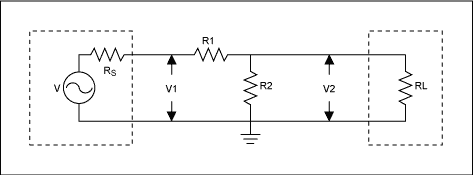Figure 3. A MLP with a high-to-low-impedance signal path.

### Voltage Loss

In CATV systems, signal level is typically measured in the voltage unit of dBmV , which is a decibel relative to 1mV. When signal level is expressed in voltage units, the voltage loss of a MLP is used. A general equation for MLP voltage loss is derived below, based on Figure 3 and assuming again that RS > RL.

By inspection of Figure 3: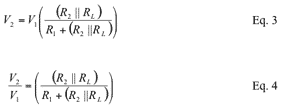Voltage loss in dB is expressed as: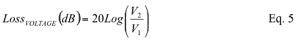Substitute Equation 4 into Equation 5: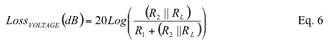Equation 6.1 is an alternate voltage-loss equation, solely in terms of RS and RL, that results from substituting Equation 1 and Equation 2 into Equation 6.### Power Loss

Power loss for a MLP is used when working with signal levels defined in units of power, for example dBm. The dBm is a decibel relative to 1mW of power. Typical test equipment measures signal level in dBm.

A general equation for MLP power loss is derived below, based on Figure 3 and Figure 4, once again assuming that RS > RL. The equations derived show that power loss consists of voltage loss plus an impedance ratio term. Power loss is calculated as the ratio of power delivered to power available .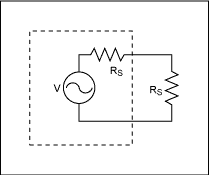Figure 4. Power available to a perfectly matched load.

Power available, PA, is the power available to a perfectly matched load, as shown in Figure 4.By inspection of Figure 3, the power delivered to the load, PD, is:V2 is expressed as: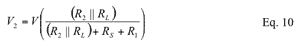Substitute Equation 10 into Equation 9: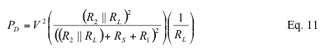Power loss in dB is expressed as: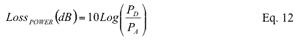Substitute Equation 8 and Equation 11 into Equation 12:By inspection of Figure 3, for a perfectly matched MLP:Substitute Equation 16 into Equation 15: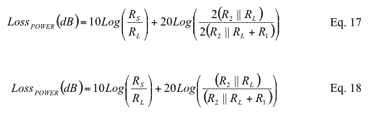Compare Equation 18 and Equation 6 to observe the difference between the power loss and voltage loss equations. Power loss is shown to consist of voltage loss plus an impedance ratio term.

Equation 18.1 is an alternate power-loss equation, solely in terms of RS and RL, that results from substituting Equation 1 and Equation 2 into Equation 18:### Low-to-High-Impedance Signal Path

To maintain consistency in notation when discussing the low-to-high-impedance signal path, Figure 5 shows RS and RL in the same circuit locations as in Figure 3, although the signal now flows through the MLP in the opposite direction.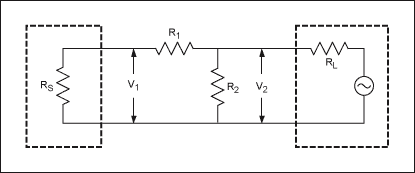Figure 5. MLP with a low-to-high-impedance signal path.

### Voltage Loss

By inspection of Figure 5:Voltage loss in dB is expressed as:Substitute Equation 20 into Equation 21:An alternate voltage-loss equation, solely in terms of RS and RL, results from substituting Equation 1 and Equation 2 into Equation 22.### Power Loss

From Figure 5, the power loss for matched source and load impedances is:Substitute Equation 20 into Equation 24.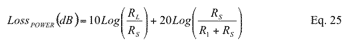An alternate power-loss equation, again only in terms of RS and RL, results from substituting Equation 1 and Equation 2 into Equation 25.Compare Equation 25 and Equation 22 to observe the difference between the power-loss and voltage-loss equations. Again for the low-to-high-impedance signal path, the power loss is shown to consist of voltage loss plus an impedance ratio term.

### 75Ω to 50Ω MLP Example

To determine the resistor values for a 75Ω to 50Ω MLP, refer to the general MLP shown in Figure 2 above. Select RS and RL to be 75Ω and 50Ω, respectively. This satisfies the assumption that RS > RL. From Equation 2: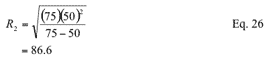From Equation 1:To determine the voltage loss of the 75Ω to 50Ω signal path, use Equation 6.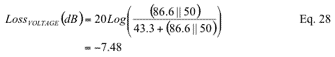To determine the power loss of the 75Ω to 50Ω signal path, use Equation 18.To determine the voltage loss of the 50Ω to 75Ω signal path, use Equation 22.To determine the power loss of the 50Ω to 75Ω signal path, use Equation 25.Note that the power loss is the same when the signal travels in either direction, while the voltage loss differs depending on signal direction. Also notice that the difference between power loss and voltage loss results from the impedance ratio term.

### Conclusion

The power loss and voltage loss of a MLP differ due to the different source and load impedances. Power loss is equal to voltage loss, plus an impedance ratio term.

The power loss is the same when the signal flows in either direction. The voltage loss, however, is larger when the signal flows from a high-impedance source to a low-impedance load.

Use voltage loss when measuring in dBmV, dBµV, or dBV, or for any voltage-related measurement. Use power loss when measuring in dBm or for any power measurement.

References

1. Bruno O. Weinschel, Reference Data for Engineers: Radio, Electronics, Computer, and Communications, Seventh Edition, Chapter 11.
2. Maxim Application Note, CATV dBm, dBmV, and dBuV Conversions.
3. Ziemer, Tranter, Principles of Communications, third edition, Appendix A, pp.759-760.
4. Krauss, Bostian, Raab, Solid State Radio Engineering, Chapter 2.